## MTK平台下Battery驱动分析及充电流程_stoic163的博客-程序员宝宝_mtk充电驱动

Kernel：

kernel-3.10\drivers\power\mediatek\

kernel-3.10\drivers\misc\mediatek\mach\mt6580\<project_name>\power\

MTK Battery框架结构图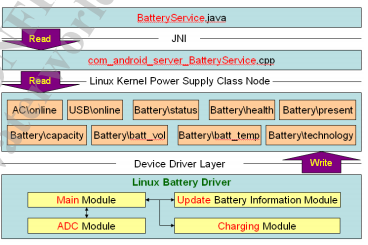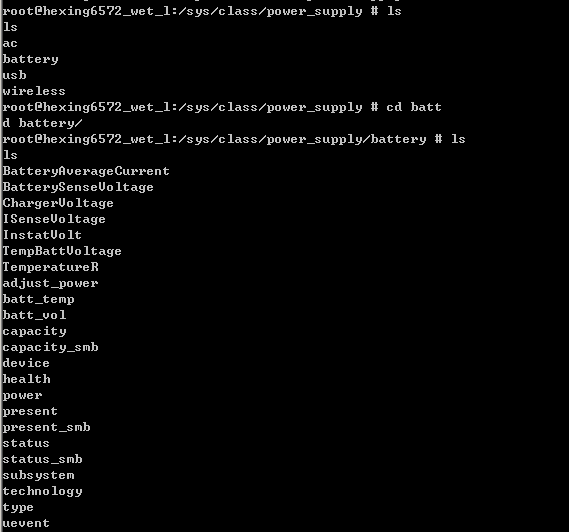Android电源管理系统
/sys/class/power_supply/ac/online //AC 电源连接状态 交流电 即电源插座
/sys/class/power_supply/usb/online //USB电源连接状态
/sys/class/power_supply/battery/status //充电状态
/sys/class/power_supply/battery/health //电池状态
/sys/class/power_supply/battery/present //使用状态
/sys/class/power_supply/battery/capacity //电池 level
/sys/class/power_supply/battery/batt_vol //电池电压
/sys/class/power_supply/battery/batt_temp //电池温度
/sys/class/power_supply/battery/technology //电池技术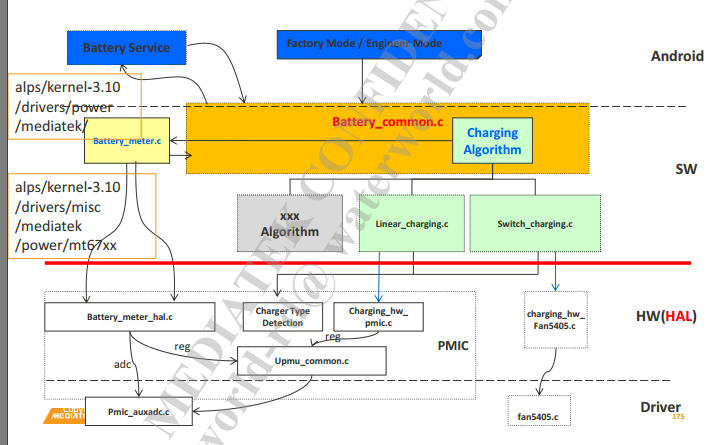battery_common.c

[objc]  view plain   copy
2. {
3.     ktime_t ktime = ktime_set(30);    /* 10s, 10* 1000 ms */
4.
5. #ifdef BATTERY_CDP_WORKAROUND
6.     if (is_usb_rdy() == KAL_FALSE) {
7.         battery_log(BAT_LOG_CRTI, "CDP, block\n");
9.         battery_log(BAT_LOG_CRTI, "CDP, free\n");
10.     } else{
11.         battery_log(BAT_LOG_CRTI, "CDP, PASS\n");
12.     }
13. #endif
14.
15.     /* Run on a process content */
16.     while (1) {
17.         mutex_lock(&bat_mutex);
18.
19.         if (((chargin_hw_init_done == KAL_TRUE) && (battery_suspended == KAL_FALSE)) || ((chargin_hw_init_done == KAL_TRUE) && (fg_wake_up_bat == KAL_TRUE)))
21.
22.         mutex_unlock(&bat_mutex);
23.
24. #ifdef FG_BAT_INT
25.         if(fg_wake_up_bat==KAL_TRUE)
26.         {
27.             wake_unlock(&battery_fg_lock);
28.             fg_wake_up_bat=KAL_FALSE;
29.             battery_log(BAT_LOG_CRTI, "unlock battery_fg_lock \n");
30.         }
31. #endif //#ifdef FG_BAT_INT
32.
33.         battery_log(BAT_LOG_FULL, "wait event\n");
34.
36.
39.         ktime = ktime_set(BAT_TASK_PERIOD, 0);  /* 10s, 10* 1000 ms 设置时间为10秒*/
40.         if (chr_wake_up_bat == KAL_TRUE && g_smartbook_update != 1/* for charger plug in/ out */
41.         {
42.             #if defined(CONFIG_MTK_DUAL_INPUT_CHARGER_SUPPORT)
43.             if (DISO_data.chr_get_diso_state) {
44.                 DISO_data.chr_get_diso_state = KAL_FALSE;
45.                 battery_charging_control(CHARGING_CMD_GET_DISO_STATE, &DISO_data);
46.             }
47.             #endif
48.
49.             g_smartbook_update = 0;
50.             #if defined(CUST_CAPACITY_OCV2CV_TRANSFORM)
51.             battery_meter_set_reset_soc(KAL_FALSE);
52.             #endif
53.             battery_meter_reset();
54.             chr_wake_up_bat = KAL_FALSE;
55.
56.             battery_log(BAT_LOG_CRTI,
57.                         "[BATTERY] Charger plug in/out, Call battery_meter_reset. (%d)\n",
58.                         BMT_status.UI_SOC);
59.         }
60.
61.     }
62.
63.     return 0;

[objc]  view plain   copy
2. {
3.     static kal_bool battery_meter_initilized = KAL_FALSE;
4.     if (battery_meter_initilized == KAL_FALSE) {
5.         battery_meter_initial();    /* move from battery_probe() to decrease booting time 第一次进该函数会进行一些初始化，如设置D0的值（初始电量，构建电池曲线等） table_init, oam_init*/
6.         BMT_status.nPercent_ZCV = battery_meter_get_battery_nPercent_zcv();
7.         battery_meter_initilized = KAL_TRUE;
8.     }
9.
10.     mt_battery_charger_detect_check();//充电器型号，是否插入等方面的检测，
11.     mt_battery_GetBatteryData();//核心函数获取电池数据
12.     if (BMT_status.charger_exist == KAL_TRUE) {
13.         check_battery_exist();
14.     }
15.     mt_battery_thermal_check();//电池温度检测以及开机mode
16.     mt_battery_notify_check();//检测电池电压，电流等
17.
18.     if (BMT_status.charger_exist == KAL_TRUE) {
19.         mt_battery_CheckBatteryStatus();//充电异常检测
20.         mt_battery_charging_algorithm();//切换充电模式 Pre_CC->CC->CV->Full
21.     }
22.
23.     mt_battery_update_status();//上报电池数据
24.     mt_kpoc_power_off_check();
25. }

mt_battery_GetBatteryData：

[objc]  view plain   copy
1. void mt_battery_GetBatteryData(void)
2. {
3.     kal_uint32 bat_vol, charger_vol, Vsense, ZCV;
4.     kal_int32 ICharging, temperature, temperatureR, temperatureV, SOC;
5.     static kal_int32 bat_sum, icharging_sum, temperature_sum;
6.     static kal_int32 batteryVoltageBuffer[BATTERY_AVERAGE_SIZE];
7.     static kal_int32 batteryCurrentBuffer[BATTERY_AVERAGE_SIZE];
8.     static kal_int32 batteryTempBuffer[BATTERY_AVERAGE_SIZE];
9.     static kal_uint8 batteryIndex = 0;
10.     static kal_int32 previous_SOC = -1;
11.
12.     bat_vol = battery_meter_get_battery_voltage(KAL_TRUE);
13.     Vsense = battery_meter_get_VSense();
14.     if( upmu_is_chr_det() == KAL_TRUE ) {
15.     ICharging = battery_meter_get_charging_current();
16.     } else {
17.         ICharging = 0;
18.     }
19.
20.     charger_vol = battery_meter_get_charger_voltage();
21.     temperature = battery_meter_get_battery_temperature();
22.     temperatureV = battery_meter_get_tempV();
23.     temperatureR = battery_meter_get_tempR(temperatureV);
24.
25.     if (bat_meter_timeout == KAL_TRUE || bat_spm_timeout == TRUE || fg_wake_up_bat== KAL_TRUE)
26.     {
27.         SOC = battery_meter_get_battery_percentage();//获取电池电量
28.         //if (bat_spm_timeout == true)
29.             //BMT_status.UI_SOC = battery_meter_get_battery_percentage();
30.
31.         bat_meter_timeout = KAL_FALSE;
32.         bat_spm_timeout = FALSE;
33.     } else {
34.         if (previous_SOC == -1)
35.             SOC = battery_meter_get_battery_percentage();
36.         else
37.             SOC = previous_SOC;
38.     }
39.
40.     ZCV = battery_meter_get_battery_zcv();
41.
42.     BMT_status.ICharging =
43.         mt_battery_average_method(BATTERY_AVG_CURRENT, &batteryCurrentBuffer, ICharging, &icharging_sum,
44.                       batteryIndex);
45.
46.
47.     if (previous_SOC == -1 && bat_vol <= batt_cust_data.v_0percent_tracking) {
48.         battery_log(BAT_LOG_CRTI,
49.                     "battery voltage too low, use ZCV to init average data.\n");
50.         BMT_status.bat_vol =
51.             mt_battery_average_method(BATTERY_AVG_VOLT, &batteryVoltageBuffer, ZCV, &bat_sum,
52.                           batteryIndex);
53.     } else {
54.         BMT_status.bat_vol =
55.             mt_battery_average_method(BATTERY_AVG_VOLT, &batteryVoltageBuffer, bat_vol, &bat_sum,
56.                           batteryIndex);
57.     }
58.
59.
60.     if (battery_cmd_thermal_test_mode == 1)
61.     {
62.         battery_log(BAT_LOG_CRTI,
63.                     "test mode , battery temperature is fixed.\n");
64.     }
65.     else
66.     {
67.     BMT_status.temperature =
68.         mt_battery_average_method(BATTERY_AVG_TEMP, &batteryTempBuffer, temperature, &temperature_sum,
69.                       batteryIndex);
70.     }
71.
72.
73.     BMT_status.Vsense = Vsense;
74.     BMT_status.charger_vol = charger_vol;
75.     BMT_status.temperatureV = temperatureV;
76.     BMT_status.temperatureR = temperatureR;
77.     BMT_status.SOC = SOC;
78.     BMT_status.ZCV = ZCV;
79.
80. #if !defined(CUST_CAPACITY_OCV2CV_TRANSFORM)
81.     if (BMT_status.charger_exist == KAL_FALSE) {
82.         if (BMT_status.SOC > previous_SOC && previous_SOC >= 0)
83.             BMT_status.SOC = previous_SOC;
84.     }
85. #endif
86.
87.     previous_SOC = BMT_status.SOC;
88.
89.     batteryIndex++;
90.     if (batteryIndex >= BATTERY_AVERAGE_SIZE)
91.         batteryIndex = 0;
92.
93.
96.
97.     battery_log(BAT_LOG_CRTI,
98.                 "AvgVbat=(%d),bat_vol=(%d),AvgI=(%d),I=(%d),VChr=(%d),AvgT=(%d),T=(%d),pre_SOC=(%d),SOC=(%d),ZCV=(%d)\n",
99.                 BMT_status.bat_vol, bat_vol, BMT_status.ICharging, ICharging,
100.                 BMT_status.charger_vol, BMT_status.temperature, temperature,
101.                 previous_SOC, BMT_status.SOC, BMT_status.ZCV);
102.
103.
104. }
battery_meter_get_battery_percentage：

[objc]  view plain   copy
1. kal_int32 battery_meter_get_battery_percentage(void)
2. {
3. //去掉了一些无效代码
4.     if (bat_is_charger_exist() == KAL_FALSE)
5.         fg_qmax_update_for_aging_flag = 1;
6.
7.
8.     oam_run();//核心函数
9.
10.     return (100 - oam_d_5);
11.
12. }
oam_run：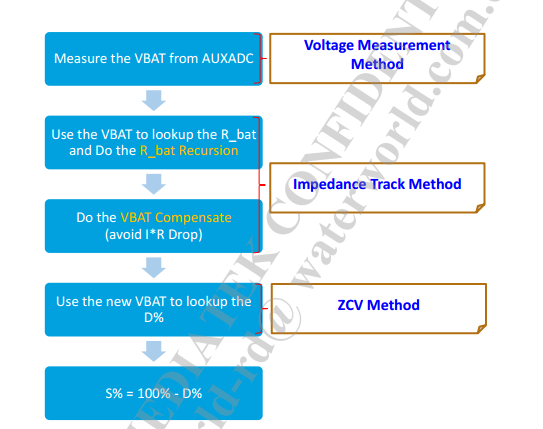[objc]  view plain   copy
1. void oam_run(void)
2. {
3.     int vol_bat = 0;
4.     /* int vol_bat_hw_ocv=0; */
5.     /* int d_hw_ocv=0; */
6.     int charging_current = 0;
7.     int ret = 0;
8.     /* kal_uint32 now_time; */
9.     struct timespec now_time;
10.     kal_int32 delta_time = 0;
11.
12.     /* now_time = rtc_read_hw_time(); */
13.     get_monotonic_boottime(&now_time);
14.
15.     /* delta_time = now_time - last_oam_run_time; */
16.     delta_time = now_time.tv_sec - last_oam_run_time.tv_sec;
17.
18.
19.     last_oam_run_time = now_time;
20.
21.     /* Reconstruct table if temp changed; */
22.     fgauge_construct_table_by_temp();
23.
24.
25.     vol_bat = 15;       /* set avg times */
27.
28.     oam_i_1 = (((oam_v_ocv_1 - vol_bat) * 1000) * 10) / oam_r_1;    /* 0.1mA  计算电流oam_v_ocv_1为上一次测得的开路电压*/
29.     oam_i_2 = (((oam_v_ocv_2 - vol_bat) * 1000) * 10) / oam_r_2;    /* 0.1mA */
30.
31.     oam_car_1 = (oam_i_1 * delta_time / 3600) + oam_car_1;  /* 0.1mAh 损失/获取 的电量 = i*t 即为放电深度，oam_car_1>0则为放电，反之则为充电*/
32.     oam_car_2 = (oam_i_2 * delta_time / 3600) + oam_car_2;  /* 0.1mAh */
33.
34.     oam_d_1 = oam_d0 + (oam_car_1 * 100 / 10) / gFG_BATT_CAPACITY_aging;//<span style="font-family: Arial, Helvetica, sans-serif;">gFG_BATT_CAPACITY_aging为电池总的容量，在oam_init的时候赋值</span>
35.
36.     if (oam_d_1 < 0)
37.         oam_d_1 = 0;
38.     if (oam_d_1 > 100)
39.         oam_d_1 = 100;
40.
41.     oam_d_2 = oam_d0 + (oam_car_2 * 100 / 10) / gFG_BATT_CAPACITY_aging;
42.     if (oam_d_2 < 0)
43.         oam_d_2 = 0;
44.     if (oam_d_2 > 100)
45.         oam_d_2 = 100;
46.
47.     oam_v_ocv_1 = vol_bat + mtk_imp_tracking(vol_bat, oam_i_25);
48.
50.     if (oam_d_3 < 0)
51.         oam_d_3 = 0;
52.     if (oam_d_3 > 100)
53.         oam_d_3 = 100;
54.
56.
59.
60.
61.     oam_d_4 = oam_d_3;
62.
63.     gFG_columb = oam_car_2 / 10;    /* mAh */
64.
65.     if ((oam_i_1 < 0) || (oam_i_2 < 0))
66.         gFG_Is_Charging = KAL_TRUE;
67.     else
68.         gFG_Is_Charging = KAL_FALSE;
69.
70.
71.     d5_count_time = 60;
72.
73.     d5_count = d5_count + delta_time;
74.     if (d5_count >= d5_count_time) {
75.         if (gFG_Is_Charging == KAL_FALSE) {
76.             if (oam_d_3 > oam_d_5) { //在放电状态下，oam_d_3大于上一次电量oam_d_5，则电池电量-1
77.                 oam_d_5 = oam_d_5 + 1;
78.             } else {
79.                 if (oam_d_4 > oam_d_5) {
80.                     oam_d_5 = oam_d_5 + 1;
81.                 }
82.             }
83.         } else {
84.             if (oam_d_5 > oam_d_3) { //<span style="font-family: Arial, Helvetica, sans-serif;">在充电状态下，oam_d_3小于上一次电量oam_d_5，则电池电量+1</span>
85.
86.                 oam_d_5 = oam_d_5 - 1;
87.             } else {
88.                 if (oam_d_4 < oam_d_5) {
89.                     oam_d_5 = oam_d_5 - 1;
90.                 }
91.             }
92.         }
93.         d5_count = 0;
94.         oam_d_3_pre = oam_d_3;
95.         oam_d_4_pre = oam_d_4;
96.     }
97.
98. }

oam_run的整个的一个流程如下图所示：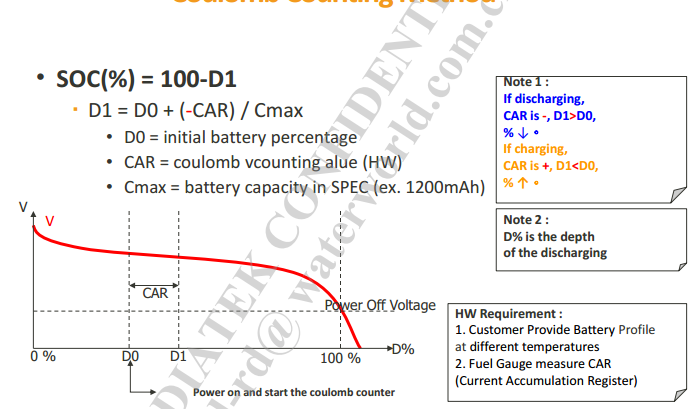MTK采用的Fuel方案获取剩余电池电量

1、电池曲线

2、充电电流的一些设置（AC_CHARGER_CURRENT，NON_STD_AC_CHARGER_CURRENT，USB_CHARGER_CURRENT等），是否是高压电池HIGH_BATTERY_VOLTAGE_SUPPORT

UI电量同步时间：SYNC_TO_REAL_TRACKING_TIME

/* Integrate with NVRAM */

//字符设备的注册，

register_chrdev_region(dev_t first,unsigned int count,char *name)
First :要分配的设备编号范围的初始值(次设备号常设为0);
Count:连续编号范围.
Name:编号相关联的设备名称. (/proc/devices);

Int alloc_chrdev_region(dev_t *dev,unsigned int firstminor,unsigned int count,char *name);
Firstminor : 通常为0;
*dev:存放返回的设备号;

Count:连续编号范围.
Name:编号相关联的设备名称. (/proc/devices);

NULL,

get_charging_control();

battery_charging_control = chr_control_interface;

status = charging_func[cmd](data);

static kal_uint32 (* const charging_func[CHARGING_CMD_NUMBER])(void *data)=
{

charging_hw_init
,charging_dump_register
,charging_enable
,charging_set_cv_voltage
,charging_get_current
,charging_set_current

......

}

/* Integrate with Android Battery Service */
ret = power_supply_register(&(dev->dev), &ac_main.psy);

BMT_status.bat_charging_state = CHR_PRE;
BMT_status.bat_in_recharging_state = KAL_FALSE;
BMT_status.bat_full = KAL_FALSE;
BMT_status.nPercent_ZCV = 0; 初始化状态

/*LOG System Set */
init_proc_log();

{

}

{
static kal_bool battery_meter_initilized = KAL_FALSE;
if (battery_meter_initilized == KAL_FALSE) {
battery_meter_initial();/* move from battery_probe() to decrease booting time */
BMT_status.nPercent_ZCV = battery_meter_get_battery_nPercent_zcv();
battery_meter_initilized = KAL_TRUE;
}

mt_battery_charger_detect_check();
mt_battery_GetBatteryData();
if (BMT_status.charger_exist == KAL_TRUE) {
check_battery_exist();
}
mt_battery_thermal_check();
mt_battery_notify_check();

if (BMT_status.charger_exist == KAL_TRUE) {
mt_battery_CheckBatteryStatus();  //检查充电状态，选择CC_PRE开始，或其他非正常状态，
mt_battery_charging_algorithm();  //真正的充电开始，如果一直在CC_PRE状态，则会反复判断是否达到

}

mt_battery_update_status();
mt_kpoc_power_off_check();
}

### 思维导图 网络拓扑图_mixboot的博客-程序员宝宝_xmind画网络拓扑图

1. 在线思维导图https://www.processon.com/

### tapestry参数_dnnlyqai的博客-程序员宝宝

tapestry如何携带两个参数啊，晕比如是一个参数的，如果还想在添加一个参数该怎样处理啊？

### Executor, ExecutorService 和 Executors 间的不同_麦晓宇的博客-程序员宝宝

java.util.concurrent.Executor, java.util.concurrent.ExecutorService, java.util.concurrent. Executors 这三者均是 Java Executor 框架的一部分，用来提供线程池的功能。因为创建和管理线程非常心累，并且操作系统通常对线程数有限制，所以建议使用线程池来并发执行任务，而不是每次请求进来时创建

### 小白学开发（iOS）OC_定义一个类（2015-07-21）_「已注销」的博客-程序员宝宝

////  main.m//  定义一个类////  Created by admin on 15/7/22.//  Copyright (c) 2015年 admin. All rights reserved.//#import /*    1. 定义一个类有三部分：        类名            要求：> 类名的首字母 必须 大写

### SSH和SSM的区别_墨羅的博客-程序员宝宝

SSH和SSM的区别加粗样式SSH是Spring+Struts2+Hibernate是目前较流行的一种Web应用程序开源集成框架。SSM是Spring+SpringMVC+MyBatis常作为数据源较简单的web项目的框架。Spring的核心功能加粗样式控制反转(IOC) &amp; 依赖注入(DI)控制反转(IOC)SpringMVC的概念加粗样式SpringMVC是Spring生态圈中的WEB-MVC框架Hibernate加粗样式是一个开放源代码的对象关系映射框架，程序员可以更加轻松的操